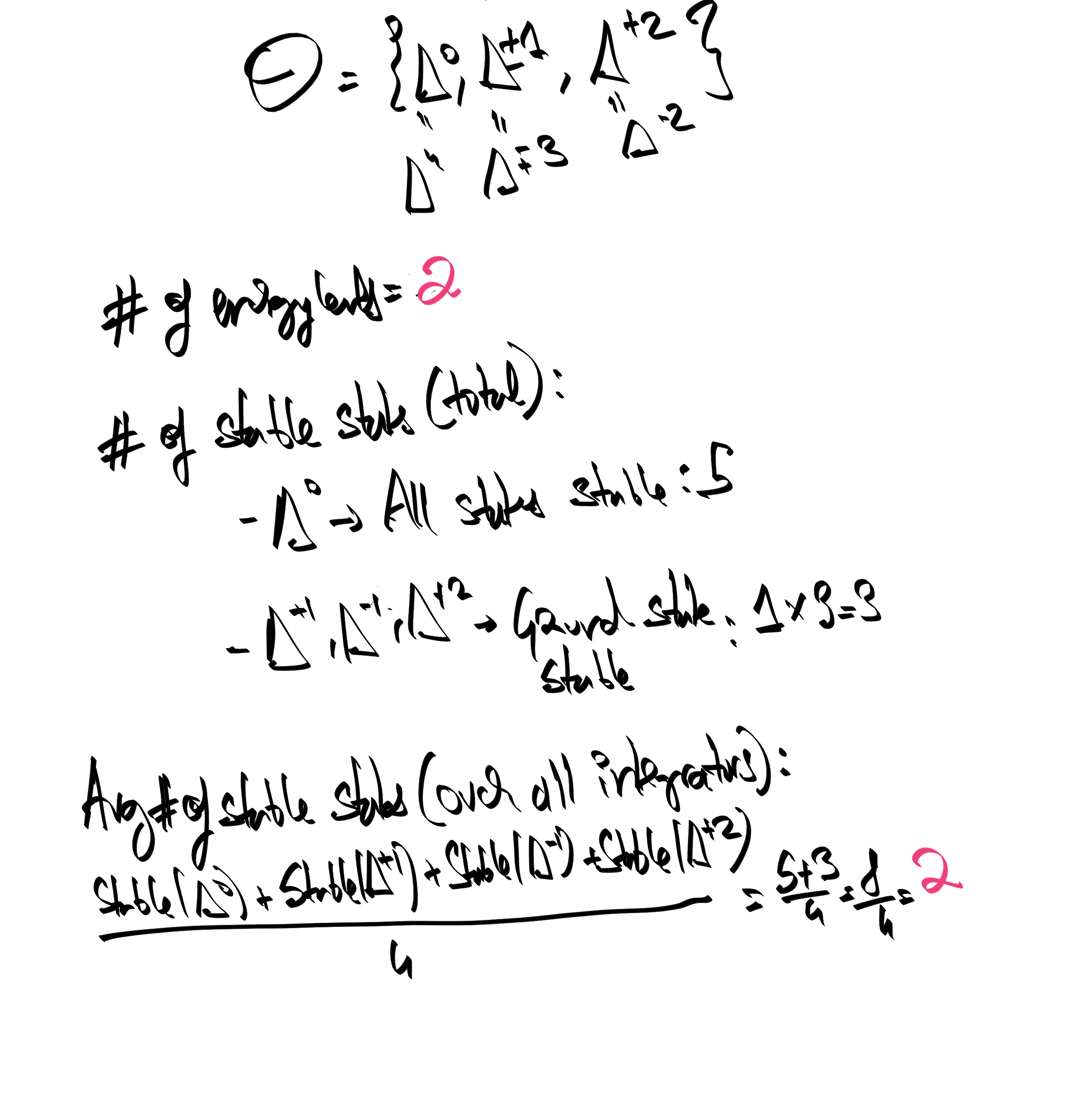## § Burnside Theorem

For a finite group $G$ acting on a set $X$, burnside's lemma equates (i) the number of equivalence classes of $X$ under $G$'s action, that is, the number of orbits of $X$ with (ii) the average number of stabilized elements for each $g \in G$. Formally, it asserts:
$|Orb(X, G)| = 1/|G|\sum_{g \in G} |Stab(g)|$

#### § As local/global principle

See that the right-hand-side measures "local fixed points" in terms of $|Stab(g)|$. The left hand side measures "global fixed points": an orbit $O \subseteq X$ is a set such that $G \cdot O = O$. So it's a sort of "global fixed point of $G$". The burnside lemms tells us that we can recover the size of the set of global fixed points (the number of orbits) by averaging the size of the set of local fixed points (the average of the sizes of the stabilizers).

#### § As space/time average

The left hand size is a time average: If we consider $X, GX, \dots G^{n}X$, we will finally be left with the images as the orbits. All other elements would have been smashed together''. On the right hand side, we are averaging the group over space. Yes, but how does one perform averaging? One needs to have a measure!

#### § A rephrasing in terms of integrators

Consider a system of a particle in a well. We consider two energy levels: that with E = 0, and E = 1. This gives us the following five states:now I want to simulate this system, like a good computer scientist. So let's write the stupdiest one possible, Δ0, that doesn't simulate anything at all, and Δ+1, which steps the system forward by a single step. These look like this:But why only these? Why privilege these time-scales? We should at least have Δ-1, for the arrow of time is fiction:We should also have coarser integrators. Hence we contemplate Δ+2 and Δ-2. Turns out these are equivalent:We can also consider Δ + 3. We also see that Δ + 4 = Δ:So in conclusion, the calculation gives us: